﻿ Mean Shift算法在目标跟踪领域的应用研究

# Mean Shift算法在目标跟踪领域的应用研究Application of Mean Shift Algorithm in Target Tracking Field

Abstract: It is still a challenge to study a real-time and accurate algorithm to solve the problem of target tracker moving constantly and being blocked in the process of movement. Mean Shift algorithm is simple in calculation and can quickly track the target. The Mean Shift algorithm principle is expounded, and two groups of experiments are carried out to track moving targets and moving targets under occlusion condition respectively. The experimental results prove that Mean Shift algorithm can realize fast and effective tracking.

1. 引言

2. Mean Shift目标跟踪算法

2.1. Mean Shift算法介绍

Mean Shift (均值漂移)是一种非参数概率密度估计的方法，一种最优的寻找概率密度极大值的梯度上升法，在解决计算机视觉底层过程中表现出了良好的鲁棒性和较高的处理速度。Mean Shift最早于1975年由Fukunaga等人在关于概率密度梯度函数的估计一文中提出 。Mean Shift算法一般指的是一个迭代的步骤，即先算出当前点的漂移均值，移动该点到其漂移均值，然后以此为新的起始点，继续移动，直到满足一定的条件结束。

2.2. Mean Shift算法原理

${M}_{h}\left(x\right)=\frac{1}{k}{\sum }_{x\in {S}_{h}}\left({x}_{i}-x\right)$ (1)

${S}_{h\left(x\right)}=P\left\{y:{\left(y-x\right)}^{\text{T}}\left(y-x\right)\le {h}^{2}\right\}$ (2)

k表示的是在有多少个样本落在了上述的多样本区域中。如图1所示，大圆所圈定的范围是 ${S}_{h}$，小圆表示落入大圆区域的样本点 ${x}_{i}$，黑点代表Mean Shift的基准偏移中心x，箭头表示样本点 ${x}_{i}$ 相对于基准点的偏移向量。在式(1)中， $\left({x}_{i}-x\right)$ 表示的是相关区域内的样本点相对于中心的偏移向量，所以计算得到的Mean Shift向量 ${M}_{h}\left(x\right)$ 是对落入区域内的所有样本点相对于中心偏移向量的平均值。由梯度的相关知识可知，非零概率密度函数的梯度总是指向概率密度函数增加最快的方向，所以平均基准点 ${M}_{h}\left(x\right)$ 会指向样本分布最多的区域，即概率密度函数的梯度方向。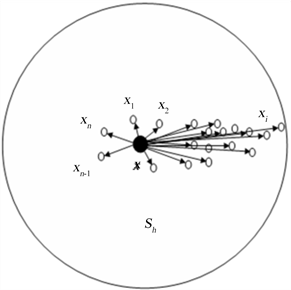Figure 1. Mean Shift schematic

2.3. Mean Shift跟踪

$\stackrel{^}{q}={\left\{{\stackrel{^}{q}}_{u}\right\}}_{u=1,\cdots ,m}{\sum }_{u=1}^{m}{\stackrel{^}{q}}_{u}=1$ (3)

$\stackrel{^}{p}={\left\{{\stackrel{^}{p}}_{u}\left(y\right)\right\}}_{u=1,\cdots ,m}{\sum }_{u=1}^{m}{\stackrel{^}{p}}_{u}\left(y\right)=1$ (4)

${\stackrel{^}{q}}_{u}=C{\sum }_{i=1}^{n}k\left({‖{x}_{i}^{*}‖}^{2}\right)\delta \left[b\left({x}_{i}^{*}\right)-u\right]C=\frac{1}{{\sum }_{i=1}^{n}k\left({‖{x}_{i}^{*}‖}^{2}\right)}$ (5)

${\stackrel{^}{p}}_{u}={C}_{k}{\sum }_{i=1}^{n}k\left({‖\frac{y-{x}_{i}}{h}‖}^{2}\right)\delta \left[b\left({x}_{i}^{\text{*}}\right)-u\right]{C}_{k}={\sum }_{i=1}^{{n}_{k}}\frac{1}{k\left({||\frac{y-{x}_{i}}{h}||}^{2}\right)}$ (6)

Bhattacharyya系数相似度测度：

$\stackrel{^}{\rho }\left(y\right)=\rho \left[\stackrel{^}{p}\left(y\right),\stackrel{^}{q}\right]={\sum }_{u=1}^{m}\sqrt{{\stackrel{^}{p}}_{u}{\stackrel{^}{q}}_{u}}$ (7)

${w}_{i}={\sum }_{u=1}^{m}\sqrt{\frac{{\stackrel{^}{q}}_{u}}{{\stackrel{^}{p}}_{u}\left({y}_{0}\right)}}\delta \left[b\left({x}_{i}\right)-u\right]$ (8)

$\stackrel{^}{{y}_{1}}=\frac{{\sum }_{i=1}^{{n}_{k}}{x}_{i}{w}_{i}g\left({‖\frac{\stackrel{^}{{y}_{0}}-{x}_{i}}{h}‖}^{2}\right)}{{\sum }_{i=1}^{{n}_{k}}{w}_{i}g\left({‖\frac{\stackrel{^}{{y}_{0}}-{x}_{i}}{h}‖}^{2}\right)}g\left(x\right)=-{k}^{\prime }\left(x\right)$ (9)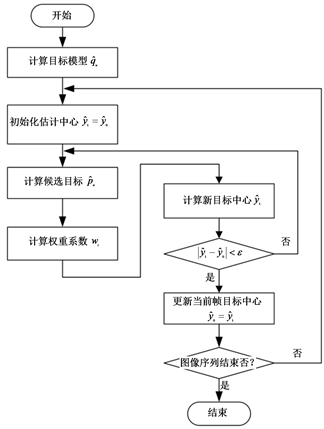Figure 2. Mean Shift tracking algorithm

3. 实验验证

3.1. 算法实现流程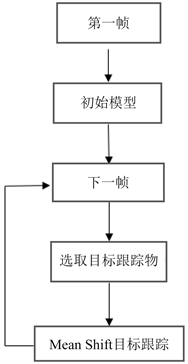Figure 3. Program flow

3.2. 实验结果

3.2.1. 运动物体的跟踪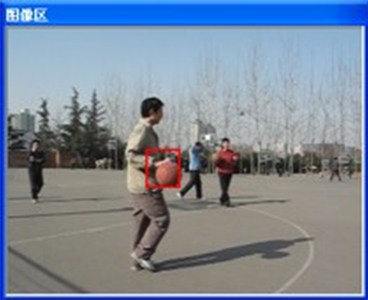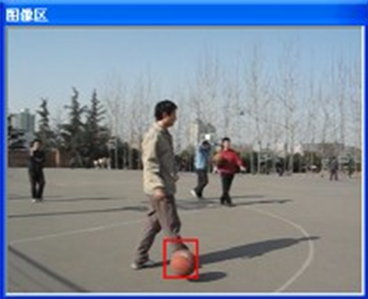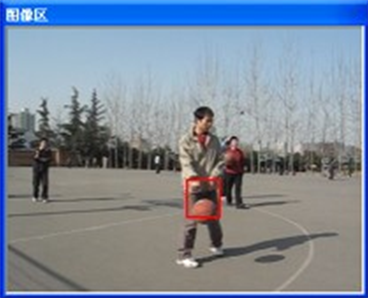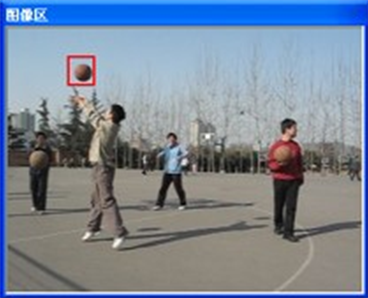Figure 4. Tracking of basketball in motion state

3.2.2. 运动物体遮挡条件下的跟踪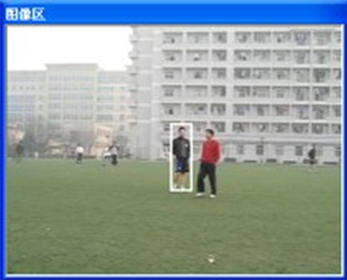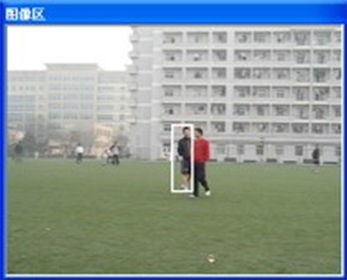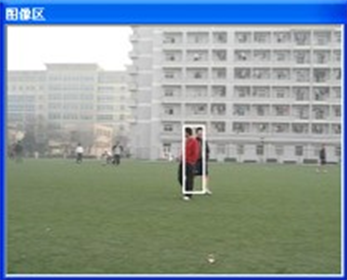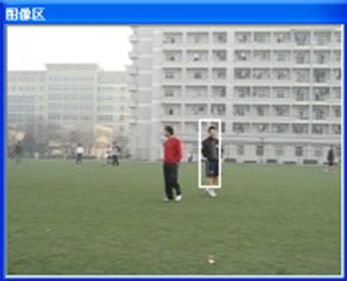Figure 5. Tracking of a moving person under occlusion

4. 结语

NOTES

*通讯作者。

 卢湖川, 李佩霞, 王栋. 目标跟踪算法综述[J]. 模式识别与人工智能, 2018, 31(1): 67-82.

 吴佳家, 高珏, 李敏, 许华虎. 基于均值漂移和卡尔曼滤波的跟踪算法研究[J]. 计算机技术与发展, 2014(1): 5-8.

 孔晓东. 智能视频监控技术研究[D].: [博士学位论文] 上海: 上海交通大学, 2008.

 赵久奋, 王明海. 红外探测阵列对固体导弹尾焰跟踪定位的研究[J]. 固体火箭技术, 2000, 23(4): 65-69.

 乐宁. 基于视觉的直升机飞行模拟及跟踪系统研究[D]: [硕士学位论文]. 南京: 南京理工大学, 2010.

 曾锐利, 李刚, 林凌. 智能交通监控系统中多目标跟踪算法[J]. 电子器件, 2007, 30(6): 185-188.

 张铁, 马琼雄. 人机交互中的人体目标跟踪算法[J]. 上海交通大学学报, 2015, 49(8): 1213-1219.

 谢达奇, 梁桥康, 孙炜, 王耀南. MeanShift聚类圆拟合算法在焊点检测中的应用[J]. 计算机仿真, 2019(9): 446-451.

 许棣华, 王志坚. 基于多任务学习的邮件过滤系统的研究[J]. 计算机技术与发展, 2010, 20(10): 137-140.

 Comaniciu, D., Ramesh, V. and Meer, P. (2002) Real-Time Tracking of Non-Rigid Objects Using Mean Shift. Proceedings IEEE Conference on Computer Vision and Pattern Recognition, CVPR 2000 (Cat. No.PR00662), Hilton Head Island, 15 June 2000.
https://doi.org/10.1109/cvpr.2000.854761

 姚放吾, 许辰铭. 基于目标质心的Meanshift跟踪算法[J]. 计算机技术与发展, 2012, 22(6): 104-106+110.

 刘巧元, 王玉茹, 张金玲, 等. 基于相关滤波器的视频跟踪方法研究进展[J]. 自动化学报, 2019, 45(2): 265-275.

 Fukunaga, K. and Hostetler, L.O. (1975) The Estimation of the Gradient of a Density Function, with Ap-plications in Pattern Recognition. IEEE Transactions on Information Theory, 21, 32-40.
https://doi.org/10.1109/tit.1975.1055330

Top